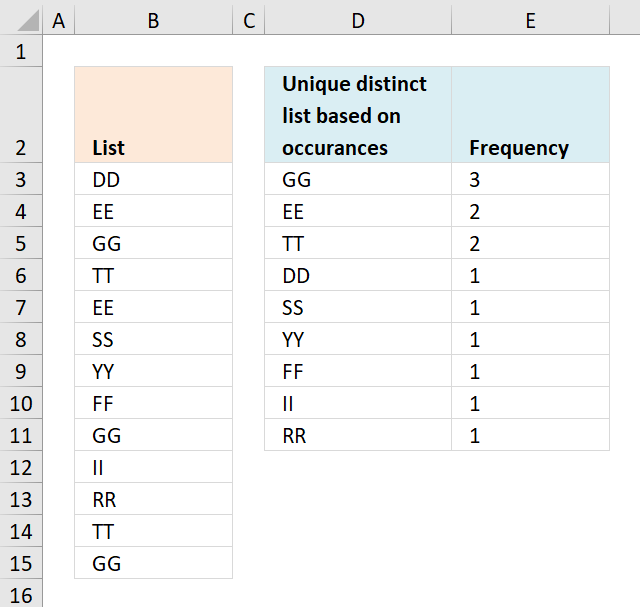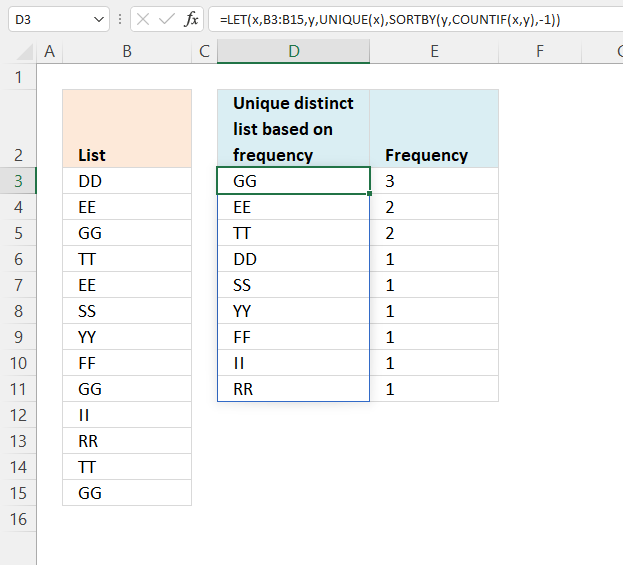Author: Oscar Cronquist Article last updated on February 17, 2023## 1. Unique distinct values sorted based on frequencyQuestion: How do I create a unique distinct list from a column sorted by occurrence?

Array formula in D3:

=INDEX(\$B\$3:\$B\$15, MATCH(IF(MAX(COUNTIF(\$B\$3:\$B\$15, \$B\$3:\$B\$15)*COUNTIF(D\$2:\$D2,\$B\$3:\$B\$15)<>1)=0, 1, MAX(COUNTIF(\$B\$3:\$B\$15, \$B\$3:\$B\$15)*IF(COUNTIF(D\$2:\$D2, \$B\$3:\$B\$15)=1,0,1))), COUNTIF(\$B\$3:\$B\$15, \$B\$3:\$B\$15)*(COUNTIF(D\$2:\$D2, \$B\$3:\$B\$15)<>1),0))

copied down as far as needed.

### How to create an array formula

1. Copy above array formula
2. Select cell D3
3. Press with left mouse button on in formula bar
4. Paste array formula in formula bar
5. Press and hold Ctrl + Shift
6. Press Enter

Formula in E3:

=COUNTIF(\$B\$3:\$B\$15, D3)

copied down as far as needed.

### Explaining formula in cell D3

#### Step 1 - Calculate frequency of each value

The COUNTIF function counts values based on a condition, in this case, multiple conditions.

COUNTIF(\$B\$3:\$B\$15, \$B\$3:\$B\$15)

becomes

COUNTIF({"DD"; "EE"; "GG"; "TT"; "EE"; "SS"; "YY"; "FF"; "GG"; "II"; "RR"; "TT"; "GG"}, {"DD"; "EE"; "GG"; "TT"; "EE"; "SS"; "YY"; "FF"; "GG"; "II"; "RR"; "TT"; "GG"})

and returns

{1; 2; 3; 2; 2; 1; 1; 1; 3; 1; 1; 2; 3}.

#### Step 2 - Prevent duplicate values

The first argument in the COUNTIF function contains an expanding cell reference. It makes sure that prior values are not taken into account again.

COUNTIF(D\$2:\$D2,\$B\$3:\$B\$15)<>1

becomes

COUNTIF("Unique distinct
list based on occurances", {"DD"; "EE"; "GG"; "TT"; "EE"; "SS"; "YY"; "FF"; "GG"; "II"; "RR"; "TT"; "GG"})<>1

becomes

{0;0;0;0;0;0;0;0;0;0;0;0;0}<>1

and returns

{TRUE; TRUE; TRUE; TRUE; TRUE; TRUE; TRUE; TRUE; TRUE; TRUE; TRUE; TRUE; TRUE}.

#### Step 3 - Multiply arrays

COUNTIF(\$B\$3:\$B\$15, \$B\$3:\$B\$15)*(COUNTIF(D\$2:\$D2,\$B\$3:\$B\$15)<>1)

becomes

{1; 2; 3; 2; 2; 1; 1; 1; 3; 1; 1; 2; 3}* {TRUE; TRUE; TRUE; TRUE; TRUE; TRUE; TRUE; TRUE; TRUE; TRUE; TRUE; TRUE; TRUE}

and returns

{1;2;3;2;2;1;1;1;3;1;1;2;3}.

#### Step 4 - Check if largest value is equal to 0 (zero)

The IF function returns 1 if number is not equal to 0 (zero) and the largest value in array if equal to zero using the MAX function.

IF(MAX(COUNTIF(\$B\$3:\$B\$15, \$B\$3:\$B\$15)*(COUNTIF(D\$2:\$D2, \$B\$3:\$B\$15)<>0))=0, 1, MAX(COUNTIF(\$B\$3:\$B\$15, \$B\$3:\$B\$15)*(COUNTIF(D\$2:\$D2, \$B\$3:\$B\$15)<>1)))

becomes

IF(MAX({1;2;3;2;2;1;1;1;3;1;1;2;3})=0, 1, MAX(COUNTIF(\$B\$3:\$B\$15, \$B\$3:\$B\$15)*(COUNTIF(D\$2:\$D2, \$B\$3:\$B\$15)<>1)))

becomes

IF(3=0, 1, MAX(COUNTIF(\$B\$3:\$B\$15, \$B\$3:\$B\$15)*(COUNTIF(D\$2:\$D2, \$B\$3:\$B\$15)<>1)))

becomes

IF(FALSE, 1, MAX(COUNTIF(\$B\$3:\$B\$15, \$B\$3:\$B\$15)*(COUNTIF(D\$2:\$D2, \$B\$3:\$B\$15)<>1)))

becomes

IF(FALSE, 1, 3)

and returns 3.

#### Step 5 - Find position in array

The MATCH function returns the position of a value in a cell range or array.

MATCH(IF(MAX(COUNTIF(\$B\$3:\$B\$15, \$B\$3:\$B\$15)*(COUNTIF(D\$2:\$D2, \$B\$3:\$B\$15)<>0))=0, 1, MAX(COUNTIF(\$B\$3:\$B\$15, \$B\$3:\$B\$15)*(COUNTIF(D\$2:\$D2, \$B\$3:\$B\$15)<>1))), COUNTIF(\$B\$3:\$B\$15, \$B\$3:\$B\$15)*(COUNTIF(D\$2:\$D2, \$B\$3:\$B\$15)<>1), 0))

becomes

MATCH(3, COUNTIF(\$B\$3:\$B\$15, \$B\$3:\$B\$15)*(COUNTIF(D\$2:\$D2, \$B\$3:\$B\$15)<>1), 0)

becomes

MATCH(3, COUNTIF(\$B\$3:\$B\$15, \$B\$3:\$B\$15)*(COUNTIF(D\$2:\$D2, \$B\$3:\$B\$15)<>1), 0)

becomes

MATCH(3, {1;2;3;2;2;1;1;1;3;1;1;2;3}, 0)

and returns 3.

#### Step 6 - Return value

The INDEX function returns a value based on a row number (and column number if needed).

INDEX(\$B\$3:\$B\$15, MATCH(IF(MAX(COUNTIF(\$B\$3:\$B\$15, \$B\$3:\$B\$15)*COUNTIF(D\$2:\$D2,\$B\$3:\$B\$15)<>1)=0, 1, MAX(COUNTIF(\$B\$3:\$B\$15, \$B\$3:\$B\$15)*IF(COUNTIF(D\$2:\$D2, \$B\$3:\$B\$15)=1,0,1))), COUNTIF(\$B\$3:\$B\$15, \$B\$3:\$B\$15)*(COUNTIF(D\$2:\$D2, \$B\$3:\$B\$15)<>1), 0))

becomes

INDEX(\$B\$3:\$B\$15, 3)

and returns "GG" in cell D3.

### Get Excel *.xlsx file

Unique-distinct-list-sorted-based-on-occurrance-in-a-column-in-excel.xlsx

## 2. Unique distinct values sorted based on frequency - Excel 365This formula works only with a single column cell range, read this article if you want to use a multi-column cell range: Extract a unique distinct list across multiple columns and rows sorted based on frequency

Excel 365 dynamic array formula in cell D3:

=LET(x,B3:B15,y,UNIQUE(x),SORTBY(y,COUNTIF(x,y),-1))

### Explaining formula

#### Step 1 - Extract unique distinct values

The UNIQUE function extracts both unique and unique distinct values and also compare columns to columns or rows to rows.

UNIQUE(array,[by_col],[exactly_once])

UNIQUE(B3:B15)

becomes

UNIQUE({"DD"; "EE"; "GG"; "TT"; "EE"; "SS"; "YY"; "FF"; "GG"; "II"; "RR"; "TT"; "GG"})

and returns

{"DD"; "EE"; "GG"; "TT"; "SS"; "YY"; "FF"; "II"; "RR"}

#### Step 2 - Count values

The COUNTIF function calculates the number of cells that meet a given condition.

COUNTIF(rangecriteria)

COUNTIF(B3:B15,UNIQUE(B3:B15))

#### Step 3 - Sort values based on count

The SORTBY function sorts values from a cell range or array based on a corresponding cell range or array.

SORTBY(array, by_array1, [sort_order1], [by_array2, sort_order2],…)

SORTBY(UNIQUE(B3:B15), COUNTIF(B3:B15,UNIQUE(B3:B15)),-1)

#### Step 4 - Shorten formula

The LET function lets you name intermediate calculation results which can shorten formulas considerably and improve performance.

LET(name1name_value1calculation_or_name2, [name_value2calculation_or_name3...])

SORTBY(UNIQUE(B3:B15), COUNTIF(B3:B15,UNIQUE(B3:B15)),-1)

x - B3:B15
y - UNIQUE(x)

LET(x,B3:B15,y,UNIQUE(x),SORTBY(y,COUNTIF(x,y),-1))